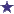# IV Compounding Question: How Many MLs Will Make Up Each Dose?

by Arlene
(Indiana)IV Compounding Question - How many MLs are each dose?

I am asked to dilute a vial of powder- c to 330mg/ml concentration. The patient needs a 600mg dose. How many mls will make up each dose?

### Comments for IV Compounding Question: How Many MLs Will Make Up Each Dose?

Average RatingRating1.8ML Makes Up Each Dose by: Zachary Given: Powder "C" is a 330mg/ml Concentation Patient needs a 600ml dose. Each dose is How many MLs? 2mls would be too much, at 660ml; and we know that 1ml (330mg) is too little. So the answer will be somewhere between 1ml and 2ml. We have final dose = 600mg dose 600mg = 330mg/ml times "X" 1ml/330mg times 600mg = "X" 1.81ml = 600mg divided by 330mg/1ml